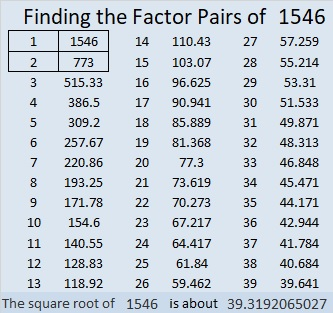# 1546 Celebrating My 7th Blogiversary

Contents

### Today’s Puzzle:

Seven years ago today at 2:11 in the morning, I published my first blog post. It featured a Find the Factors 1 – 10 puzzle with only perfect square clues. In honor of that first post, I publish this puzzle, also with only perfect square clues. This one looks like an exclamation point because I have enjoyed creating puzzles and writing these posts for you so much these seven years!How do you solve the puzzle? Use logic to write the numbers from 1 to 10 in both the first column and the top row so that the puzzle functions like a multiplication table.

### Factors of 1546:

• 1546 is a composite number.
• Prime factorization: 1546 = 2 × 773.
• 1546 has no exponents greater than 1 in its prime factorization, so √1546 cannot be simplified.
• The exponents in the prime factorization are 1 and 1. Adding one to each exponent and multiplying we get (1 + 1)(1 + 1) = 2 × 2 = 4. Therefore 1546 has exactly 4 factors.
• The factors of 1546 are outlined with their factor pair partners in the graphic below.### More about the Number 1546:

1546 is the sum of two squares:
39² + 5² = 1546.

1546 is the hypotenuse of a Pythagorean triple:
390-1496-1546, calculated from 2(39)(5), 39² – 5², 39² + 5².
It is also 2 times (195-748-773)

This site uses Akismet to reduce spam. Learn how your comment data is processed.